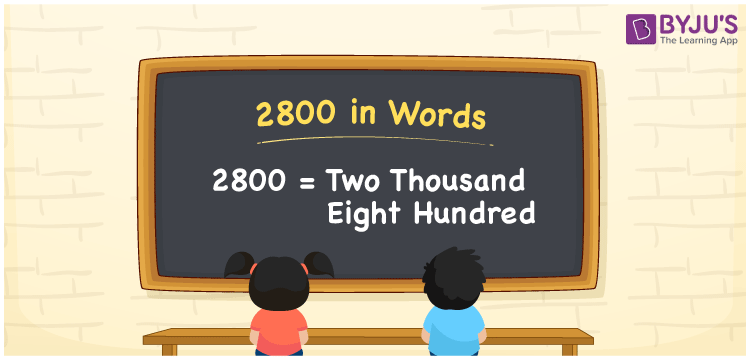# 2800 in words

2800 in words is written as Two Thousand Eight Hundred. In the number 2800, 2 represents the place value of thousand and 8 has the place of hundred. The article on Place Value will get you more details. The number 2800 is used in expressions that relate to count, money, distance, length and others. Let us consider an example for the number 2800. “A new library opened with Two Thousand Eight Hundred Books.” Another example, “A tiny story book has 2800 words in it.”

 2800 in words Two Thousand Eight Hundred Two Thousand Eight Hundred in Numbers 2800

## 2800 in English Words## How to Write 2800 in Words?

We can convert 2800 to words using a place value chart. The number 2800 has 4 digits, so let’s make a chart that shows the place value up to 4 digits.

 Thousands Hundreds Tens Ones 2 8 0 0

Thus, we can write the expanded form as:

2 × Thousand + 8 × Hundred + 0 × Ten + 0 × One

= 2 × 1000 + 8 × 100 + 0 × 10 + 0 × 1

= 2800

= Two Thousand Eight Hundred

2800 is the natural number that is succeeded by 2799 and preceded by 2801.

2800 in words – Two Thousand Eight Hundred.

Is 2800 an odd number? – No.

Is 2800 an even number? – Yes.

Is 2800 a perfect square number? – No.

Is 2800 a perfect cube number? – No.

Is 2800 a prime number? – No.

Is 2800 a composite number? – Yes.

## Solved Example

1. Write the number 2800 in expanded form

Solution: 2 × 1000 + 8 × 100 + 0 × 10 + 0 × 1

We can write 2800 = 2000 + 800 + 0 + 0

= 2 × 1000 + 8 × 100 + 0 × 10 + 0 × 1

## Frequently Asked Questions on 2800 in words

Q1

### How to write 2800 in words?

2800 in words is written as Two Thousand Eight Hundred.
Q2

### State True or False. 2800 is divisible by 2?

True. 2800 is divisible by 2.
Q3

### Is 2800 divisible by 10?

Yes. 2800 is divisible by 10. It is also divisible by 2, 4, 5, 10 and 100.